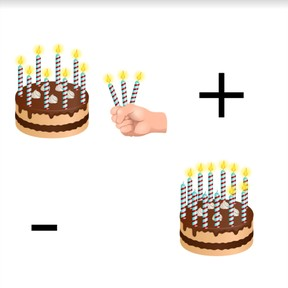Recognizing addition and subtraction situations to 20

# Recognizing addition and subtraction situations to 208,000 schools use Gynzy92,000 teachers use Gynzy1,600,000 students use Gynzy

## General

Students learn to recognize addition or subtraction situations by using an image.

1.OA.A.1

## Relevance

Discuss with students that it is useful to be able to recognize if something is being added or taken away to a situation. You learn how math problems are formed and can learn to add and subtract.

## Introduction

Show the image of the umbrellas on the interactive whiteboard and ask students to count how many there are. Remind students that they can mark off the umbrellas as they count them, to make it easier. Ask the students to choose from the given numbers. Then show students the image of the boots and again, ask them to count how many there are.

## Development

Using the chocolates shown on the interactive whiteboard, show the students an 'adding to' situation. There are chocolates on the tray, and chocolates that are added to the tray. that still need to be filled. First count the chocolates on the tray and then the chocolates that are being added to the tray. Discuss all the variations with the chocolates and show the accompanying math problem to each image.
Then show students a 'taking away' situation. Use the chocolates again, show the chocolates on the tray and the empty chocolate wrappers. First count how many chocolates there are in total, and then count how many chocolate wrappers there are. Discuss all the variations with the chocolates and show the accompanying math problem to each image.
Next discuss how to find the math problem using the bowls. Determine the problem by first counting how many bowls are stacked, which is the first number of the addition problem, then counting how many bowls are being dried (waiting to be added), which is the second number of the addition problem, and then counting those two numbers together. Discuss a situation in which things are taken away using the blocks of cheese. The first number is the total number of blocks of cheese on the party tray. The second number is the number of blocks of cheese that were eaten. Practice more adding to situations with the pencils and taking away problems with the ice cubes.
Tell students that now that you can determine what math problem is being represented by an image, you can also find the total or difference. Using the bowls, show students that you can solve an adding to problem by counting the numbers together to find the total. Using the blocks of cheese, show students that you can solve a taking away problem by taking away the second number from the first to find the difference. To practice both addition and subtraction, show students a set of adding to and taking from problems to solve. Ask students to first determine what the math problem is, and then to solve. Have students explain their strategy.

Check that students are able to recognize addition or subtraction problems using an image by asking the following questions:
- How do you know which numbers to use in a math problem?
- What do you do when you want to find the total of a set of numbers?
- What do you do if you want to find the difference between a set of numbers?

## Guided practice

Students are first given problems in which they are asked to recognize or write the math problem that matches the given image. They are then asked to write and solve the math problem represented by the given image.

## Closing

Remind students that it is important to be able to recognize if a situation is addition or subtraction so that they can learn to determine how many they have, or have left. Then use the interactive whiteboard to show two statements. Two students are shown saying which math problem matches the given image, the class must determine which student is correct. Ask students to explain how they determined the math problem represented by the image. Then ask students to draw an image that represents an addition or subtraction problem, and to write two statements to accompany their drawing. They swap papers with a classmate and they must determine which statement is correct.

## Teaching tips

Students who have difficulty adding or subtracting using an image can be supported by the use of MAB blocks or other manipulatives. To practice addition, have them make two groups of blocks, one for each number. Then count the blocks on the left, and use the blocks on the right to count on. To practice subtraction, have them make one group of blocks for the first number. Then they should take away one block at a time until they create a pile that is equal to the second number. The amount left is the difference.

## Instruction materials

Optional: MAB blocks or other manipulatives.

### The online teaching platform for interactive whiteboards and displays in schools

• Save time building lessons

• Manage the classroom more efficiently

• Increase student engagement